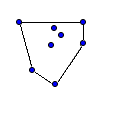CGAL 5.3 - 2D Convex Hulls and Extreme Points
2D Convex Hulls and Extreme Points ReferenceSusan Hert and Stefan Schirra
This package provides functions for computing convex hulls in two dimensions as well as functions for checking if sets of points are strongly convex are not. There are also a number of functions described for computing particular extreme points and subsequences of hull points, such as the lower and upper hull of a set of points.
Introduced in: CGAL 1.0
BibTeX: cgal:hs-chep2-21a
Windows Demo: See Bounding Volumes
Common Demo Dlls: dlls

A subset $$S \subseteq \mathbb{R}^2$$ is convex if for any two points p and q in the set the line segment with endpoints p and q is contained in $$S$$. The convex hull of a set $$S$$ is the smallest convex set containing $$S$$. The convex hull of a set of points P is a convex polygon with vertices in P. A point in P is an extreme point (with respect to P) if it is a vertex of the convex hull of P.

CGAL provides functions for computing convex hulls in two dimensions as well as functions for testing if a given set of points is strongly convex or not. There are also a number of functions available for computing particular extreme points in 2D and subsequences of the hull points, such as the lower hull or upper hull of a set of points.

## Assertions

The assertion flags for the convex hull and extreme point algorithms use CH in their names (e.g., CGAL_CH_NO_POSTCONDITIONS). For the convex hull algorithms, the postcondition check tests only convexity (if not disabled), but not containment of the input points in the polygon or polyhedron defined by the output points. The latter is considered an expensive checking and can be enabled by defining CGAL_CH_CHECK_EXPENSIVE.

## Concepts

• ConvexHullTraits_2

## Traits Classes

• CGAL::Convex_hull_constructive_traits_2<R>
• CGAL::Convex_hull_traits_2<R>
• CGAL::Convex_hull_traits_adapter_2<R,P>

## Convex Hull Functions

• CGAL::ch_akl_toussaint()
• CGAL::ch_bykat()
• CGAL::ch_eddy()
• CGAL::ch_graham_andrew()
• CGAL::ch_jarvis()
• CGAL::ch_melkman()
• CGAL::convex_hull_2()

## Convexity Checking Functions

• CGAL::is_ccw_strongly_convex_2()
• CGAL::is_cw_strongly_convex_2()

## Hull Subsequence Functions

• CGAL::ch_graham_andrew_scan()
• CGAL::ch_jarvis_march()
• CGAL::lower_hull_points_2()
• CGAL::upper_hull_points_2()

## Extreme Point Functions

• CGAL::ch_e_point()
• CGAL::ch_nswe_point()
• CGAL::ch_n_point()
• CGAL::ch_ns_point()
• CGAL::ch_s_point()
• CGAL::ch_w_point()
• CGAL::ch_we_point()

## Modules

Concepts

Traits Classes

Convex Hull Functions

Convexity Checking

Hull Subsequence Functions

Extreme Point Functions